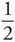# AP Physics 1: Oscillations Multiple-Choice Practice Questions

### Test Information9 questions18 minutes

1. A block attached to an ideal spring undergoes simple harmonic motion. The acceleration of the block has its maximum magnitude at the point where

2. A block attached to an ideal spring undergoes simple harmonic motion about its equilibrium position (x = 0) with amplitude A. What fraction of the total energy is in the form of kinetic energy when the block is at position x =A ?

3. A student measures the maximum speed of a block undergoing simple harmonic oscillations of amplitude A on the end of an ideal spring. If the block is replaced by one with twice its mass but the amplitude of its oscillations remains the same, then the maximum speed of the block will

4. A spring-block simple harmonic oscillator is set up so that the oscillations are vertical. The period of the motion is T. If the spring and block are taken to the surface of the Moon, where the gravitational acceleration is 1/6 of its value here, then the vertical oscillations will have a period of

5. A linear spring of force constant k is used in a physics lab experiment. A block of mass m is attached to the spring and the resulting frequency, f, of the simple harmonic oscillations is measured. Blocks of various masses are used in different trials, and in each case, the corresponding frequency is measured and recorded. If f2 is plotted versus 1/m, the graph will be a straight line with slope

6. A simple pendulum swings about the vertical equilibrium position with a maximum angular displacement of 5° and period T. If the same pendulum is given a maximum angular displacement of 10°, then which of the following best gives the period of the oscillations?

7. A block with a mass of 20 kg is attached to a spring with a force constant k = 50 N/m. What is the magnitude of the acceleration of the block when the spring is stretched 4 m from its equilibrium position?

8. A block with a mass of 10 kg connected to a spring oscillates back and forth with an amplitude of 2 m. What is the approximate period of the block if it has a speed of 4 m/s when it passes through its equilibrium point?

9. A block with a mass of 4 kg is attached to a spring on the wall that oscillates back and forth with a frequency of 4 Hz and an amplitude of 3 m. What would the frequency be if the block were replaced by one with one-fourth the mass and the amplitude of the block is increased to 9 m ?